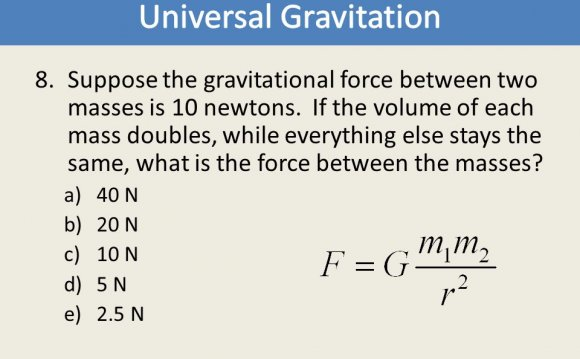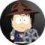# Gravitational force between two masses

December 11, 2015In order to calculate the gravitational force between two objects with masses of m1 and m2, the equation is:

where G is the gravitational constant (6.67E-11 m3 s-2 kg-1), r is the distance between the two objects, and F is the magnitude of the force between the objects.

So let's try to solve this problem: Suppose I own two planets. I would like to send resources between my planets, but I need to know the force generated between them in order to correctly launch my freight shuttles. One of my planets weighs 6.0E24 kg (the mass of the Earth) while the other weighs 4.9E24 kg (the mass of Venus). The distance between the planets is 4.1E10 m (the distance between Earth and Venus).

Electromagnetic force

I realize that I have gone out of the order in which these forces were introduced, but the calculations for EMF are far simpler than the calculations for either the weak or strong force. So I will explain the calculations for EMF first.

The force of attraction (or repulsion) of two particles can be calculated using Coulomb’s law. The law states:

where k is 8.99E9(Nm2/C2), q(sub)a and q(sub)b are the charges of particles a and b, and r is the distance between the particles.

To put this equation to work, consider the following problem. What is the electric force between 2 up quarks separated by 1.0E-16 meters? This is a typical separation inside a proton. Given: The charge of an up quark is (2/3)e.

In order to solve this equation we need to know the value of k, both q’s, and r. k is the constant listed above. q(sub)a and q(sub)b = (2/3)*e, and r = 1.0E-16 m. Now we can simply plug and chug.

The Strong Force

The strong force is, by far, the most difficult force to calculate. The calculation of the strong force using QCD (quantum chromodynamics) started in the 1950’s when scientists first began to notice that there were far more fundamental particles than the proton, neutron, and electron. Scientists found that there were far smaller particles that formed protons and neutrons. They called these particles “quarks.” (WHY QUARKS?) Scientists first believed that all quarks were the same. Through further experimentation and calculation, this was proven to be false by the Pauli Exclusion Prinicple. Throughout the 1970’s, physicists were bogged down by the extreme amount of math involved in determining the force between two quarks.

Murray Gell-Mann found that quarks contained a property separate from charge. This property was called “color.” This color charge was found when Gell-Mann tried to explain the existence of a particle containing three up quarks (according to the Pauli exclusion principle this is impossible). More about the history of QCD will be explained later. For now, let us look at the equations associated with QCD. Press "play" in the animation below to find out more about the equations associated with the strong force. Then follow the links below to read more about the strong force.

Source: home.fnal.gov
##### INTERESTING VIDEOMass Transfer Between Two Containers (Interactive)What Happens to the Gravitational Force Between 2 Planets ...Relationship between Gravitational Force and mass / distance.

##### INTERESTING FACTS